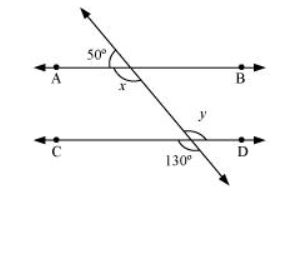# In the given figure, find the values of x and y and then show that AB || CD.Solution:

It can be observed that,

$50^{\circ}+x=180^{\circ}($ Linear pair $)$

$x=130^{\circ} \ldots$(1)

Also, $y=130^{\circ}$ (Vertically opposite angles)

As $x$ and $y$ are alternate interior angles for lines $A B$ and $C D$ and also measures of these angles are equal to each other, therefore, line $A B \|$ CD.